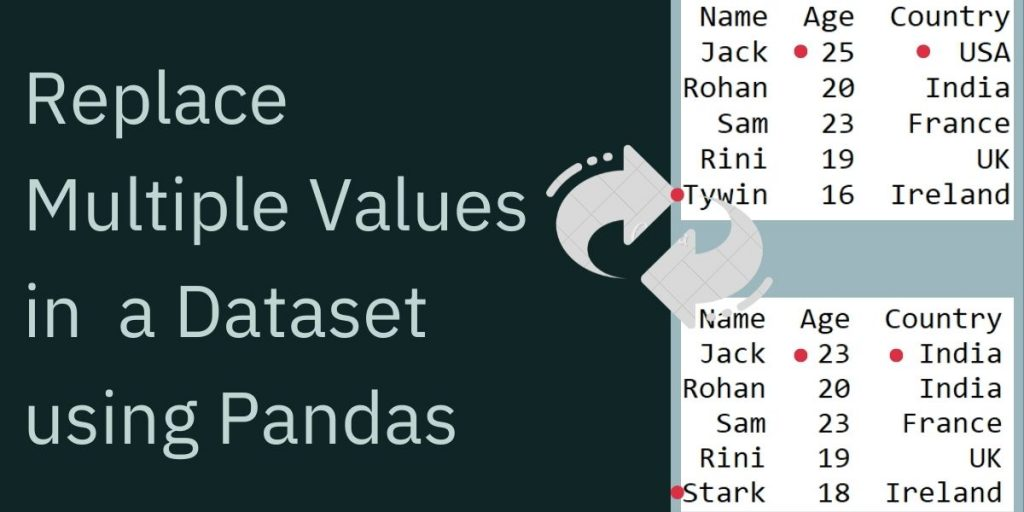# Replace Multiple Values in a Dataset using PandasIn this tutorial, we’ll see how to change multiple values in a dataset using the pandas replace() method. Pandas is a python library for data manipulation and analysis which provides a wide range of features to make the dataset usable.

## Replace Multiple Values in a Dataset

Follow the below step-by-step tutorial to replace multiple values in a dataset using the pandas library.

### 1. Import Pandas

Start by importing Pandas into your code.

```import pandas as pd
```

### 2. Sample Data

We will use the following dataset as an example and implement it in a Pandas DataFrame where ‘columns’ represent the column heading of the Dataset, as defined in the DataFrame.

```import pandas as pd
data = pd.DataFrame([
['Jack',25,'USA'],
['Rohan',20,'India'],
['Sam',23,'France'],
['Rini',19,'UK'],
['Tywin',16,'Ireland']],

columns=['Name','Age', 'Country'])
```

When the program is executed, and the DataFrame is printed using the print method, the above code results in the following output – having 3 columns ‘Name’, ‘Age’, ‘Country’.

```print (data)
```Sample Data To Replace Multiple Values

### 3. Replacing Values using replace() Method

Using the replace() method in pandas replaces a specified value with another specified value. In our sample dataset created we wish to change the USA to India in the ‘Country’ column:

```new_data = data.replace({'Country':{'USA':'India'}})

print (new_data)
```

Now, if we try to replace multiple values at once in a DataFrame, as like 25 to 23, also 16 to 18 in the Age column and ‘Tywin’ to ‘Stark’ in the Name column, here’s what the code would look like:

```updated_data = new_data.replace({'Age': {25:23, 16:18}, 'Name':{'Tywin':'Stark'}})

print(updated_data)
```

Final output after changing the desired values:

## Complete Code to Replace Multiple Values in a Dataframe

Try the code below to see how the replace() method in Python works.

```import pandas as pd
data = pd.DataFrame([
['Jack',25,'USA'],
['Rohan',20,'India'],
['Sam',23,'France'],
['Rini',19,'UK'],
['Tywin',16,'Ireland']],

columns=['Name','Age', 'Country'])
print (data)

print('\n')

new_data = data.replace({'Country':{'USA':'India'}})

print (new_data)

updated_data = new_data.replace({'Age': {25:23, 16:18}, 'Name':{'Tywin':'Stark'}})

print('\n')
print(updated_data)

```

## Conclusion

That’s It! for this tutorial. Hope you have learned well how to replace multiple values in a Pandas DataFrame in Python. Stay tuned to AskPython for more such tutorials.